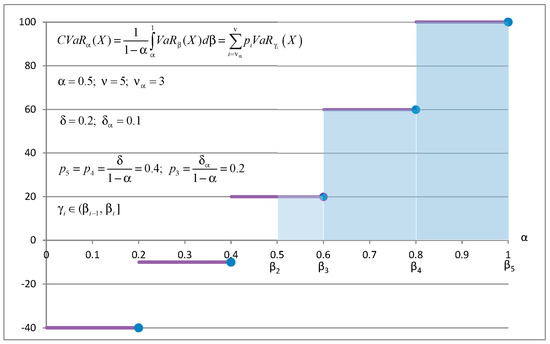Next Article in Journal
The Outperformance Probability of Mutual Funds
Previous Article in Journal
Bank Competition, Foreign Bank Entry, and Risk-Taking Behavior: Cross Country Evidence
Previous Article in Special Issue
Examination and Modification of Multi-Factor Model in Explaining Stock Excess Return with Hybrid Approach in Empirical Study of Chinese Stock Market

Article Menu
Metrics 0

## Export Article

Open AccessArticle

# CVaR Regression Based on the Relation between CVaR and Mixed-Quantile Quadrangles

1
V.M. Glushkov Institute of Cybernetics, 40, pr. Akademika Glushkova, 03187 Kyiv, Ukraine
2
Applied Mathematics & Statistics, Stony Brook University, B-148 Math Tower, Stony Brook, NY 11794, USA
*
Author to whom correspondence should be addressed.
J. Risk Financial Manag. 2019, 12(3), 107; https://doi.org/10.3390/jrfm12030107
Received: 16 May 2019 / Revised: 19 June 2019 / Accepted: 20 June 2019 / Published: 26 June 2019
(This article belongs to the Special Issue Mathematical Finance with Applications)
|
PDF [1131 KB, uploaded 8 July 2019]
|

# Abstract

A popular risk measure, conditional value-at-risk (CVaR), is called expected shortfall (ES) in financial applications. The research presented involved developing algorithms for the implementation of linear regression for estimating CVaR as a function of some factors. Such regression is called CVaR (superquantile) regression. The main statement of this paper is: CVaR linear regression can be reduced to minimizing the Rockafellar error function with linear programming. The theoretical basis for the analysis is established with the quadrangle theory of risk functions. We derived relationships between elements of CVaR quadrangle and mixed-quantile quadrangle for discrete distributions with equally probable atoms. The deviation in the CVaR quadrangle is an integral. We present two equivalent variants of discretization of this integral, which resulted in two sets of parameters for the mixed-quantile quadrangle. For the first set of parameters, the minimization of error from the CVaR quadrangle is equivalent to the minimization of the Rockafellar error from the mixed-quantile quadrangle. Alternatively, a two-stage procedure based on the decomposition theorem can be used for CVaR linear regression with both sets of parameters. This procedure is valid because the deviation in the mixed-quantile quadrangle (called mixed CVaR deviation) coincides with the deviation in the CVaR quadrangle for both sets of parameters. We illustrated theoretical results with a case study demonstrating the numerical efficiency of the suggested approach. The case study codes, data, and results are posted on the website. The case study was done with the Portfolio Safeguard (PSG) optimization package, which has precoded risk, deviation, and error functions for the considered quadrangles. View Full-Text
FiguresFigure 1

This is an open access article distributed under the Creative Commons Attribution License which permits unrestricted use, distribution, and reproduction in any medium, provided the original work is properly cited (CC BY 4.0).# Share & Cite This Article

MDPI and ACS Style

Golodnikov, A.; Kuzmenko, V.; Uryasev, S. CVaR Regression Based on the Relation between CVaR and Mixed-Quantile Quadrangles. J. Risk Financial Manag. 2019, 12, 107.

Note that from the first issue of 2016, MDPI journals use article numbers instead of page numbers. See further details here.

1

# Comments

[Return to top]
J. Risk Financial Manag. EISSN 1911-8074 Published by MDPI AG, Basel, Switzerland RSS E-Mail Table of Contents Alert
Back to Top